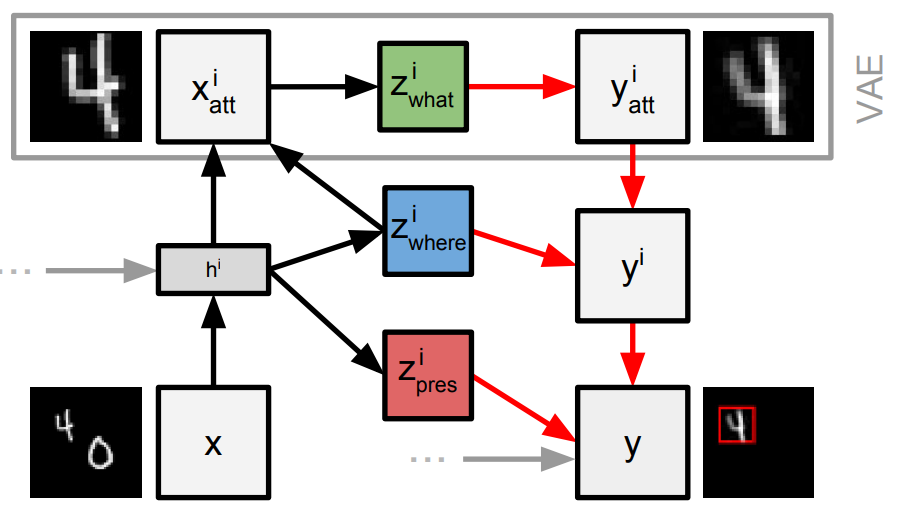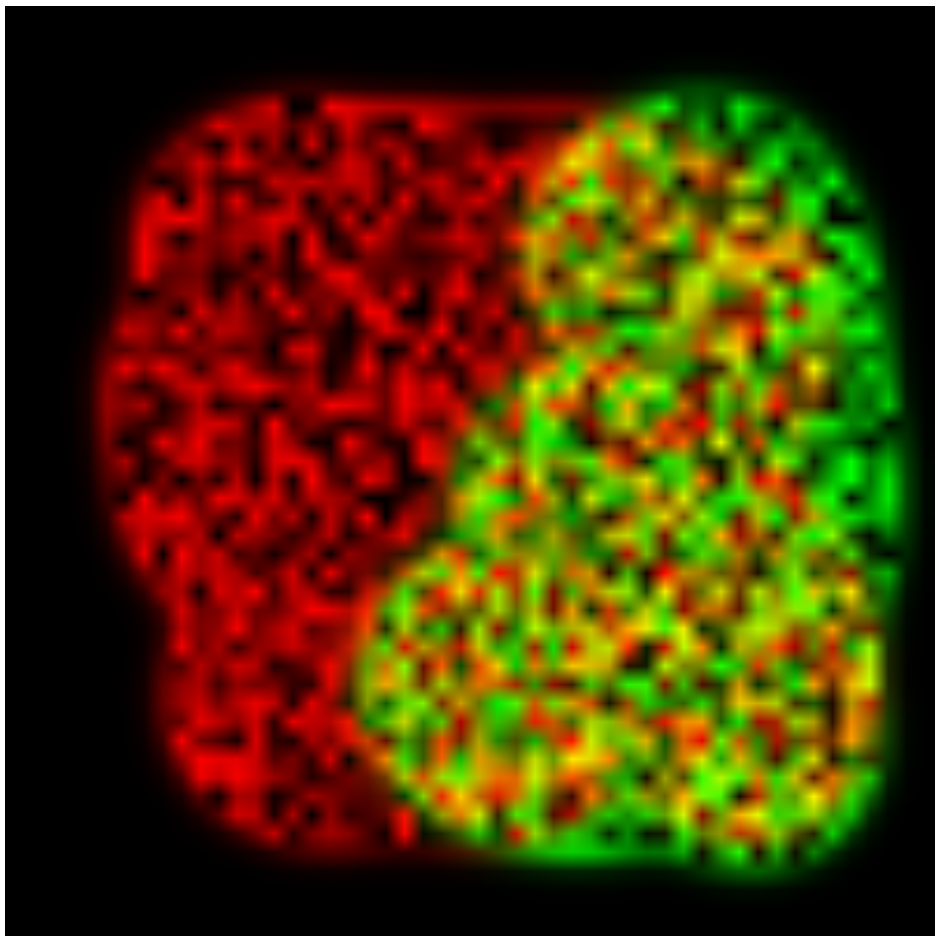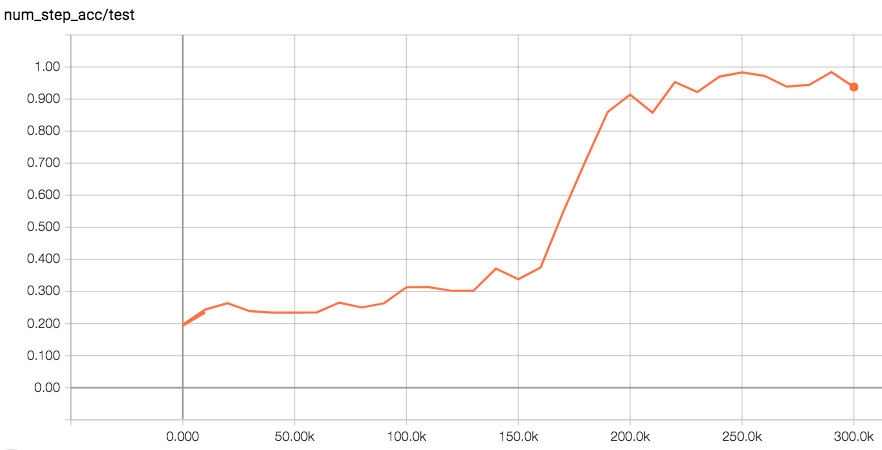Variational Autoencoders (VAEs) are getting more and more popular in the Machine Learning community. While the formulation is more involved then that of a typical feed-forward neural network, VAEs have a lot of added benefits. I’ve been recently playing with one of the more complicated VAE models: Attend, Infer, Repeat (AIR) by Ali Eslami et. al. from DeepMind, and I must say it’s really cool. In this blog post, I will describe the model and break it down into simple components. We will also cover parts of the implementation and some issues I had while implementing it. The full implementation is available here.

# What does AIR do?

AIR aims to reconstruct an image, but instead of doing it in a single shot, it focuses on interesting image parts one-by-one. The figure below demonstrates AIR’s inner workings. It takes a look at the image, figures out how many interesting parts there are and where they are in the image. It then reconstructs them by painting one-part-at-a-time onto a blank canvas. AIR takes a look at the image, figures out how many interesting parts there are, and reconstructs it by painting one-part-at-a-time onto a blank canvas. Sounds easy enough? Well, it’s not, and for two reasons:

• It’s completely unsupervised,
• It takes a variable yet discrete number of steps.

The first one is tricky, because we don’t really know how to define an object or an interesting part (more on this later). The second leads to discrete latent variables, which are not-that-easy to deal with when computing gradients.Let’s go back to the figure. AIR is called Attend, Infer, Repeat for a reason:

• It attends to a part of an image using spatial transformers, effectively cropping it,
• then it infers the latent variables best describing the crop,
• and finally it repeats the procedure for the rest of the image.

Technically, the order is different, because it has to infer presence of an object and its location before attending to it; and the name describes only the inference process, not reconstruction.

What’s beautiful, is that we get a variable-length representation of the image: the more complicated the image is, the longer the representation will be. What’s even better, is that we know that each piece of description is tied to a particular location (and hopefully an object), which allows explicit reasoning about objects and relations between them.

# Results

Measuring performance of generative models is always tricky, and I’d recommend this paper for a discussion. Here are some plots similar to the ones reported by the AIR paper. The first row of the topmost figure shows the input images, rows 2-4 are reconstructions at steps 1, 2 and 3 (with marked location of the attention glimpse in red, if it exists). Rows 5-7 are the reconstructed image crops, and above each crop is the probability of executing 1, 2 or 3 steps. If the reconstructed crop is black and there is “0 with …” written above it, it means that this step was not used (3rd step is never used, hence the last row is black). Click on the image for a higher-resolution view.

At every time-step, AIR chooses where to look in the image. The image on the left hand-side visualises the localisation policy of the spatial transformer, with red corresponding to the first step and green to the second. We see that the scanning policy is spatial with the majority of first steps located on the left hand-side of the image. The plot on the right hand-side is the counting accuracy on the validation set while training for 300k iterations, evaluated every 10k iterations.AIR can reach almost 100% accuracy in counting objects, but this outcome does heavily depend on initialisation. Very often (80% of the time) the model converges to either zero or the maximum number of steps and fails to converge to the preferred solution.

# Why and how does it work?

Like every VAE, AIR is trained by maximising the evidence lower bound (ELBO) $$\mathcal{L}$$ on the log probability of the data:

\begin{align*} \log p(x) &= \mathcal{L}(\theta, \phi) + KL(q_\phi(z \mid x) \mid\mid p(z \mid x)),\\ \mathcal{L}(\theta, \phi) &= \mathbb{E}_{q_\phi(z)} [\log p_\theta(x \mid z)]] - KL(q_\phi(z\mid x) \mid\mid p(z)). \end{align*}

The first term of the ELBO is a probabilistic analog of the reconstruction error and the second term acts as a regulariser. For AIR, the second term tries to keep the number of steps low, but it’s also forcing the latent encoding of each image part to be as short as possible.

Short encoding means that the model has to focus on parts of the image that can be explained with relatively few variables. It turns out that we can define an object as an image patch, where pixel correlations within that patch are strong, but the correlation between pixels inside and outside of that patch is weak. We can also assume that pixels belonging to two different objects have very low correlation (as long as the two objects appear independently of each other). That means that explaining even small parts of two different objects at the same time leads to potentially longer encoding than explaining one (potentially big) object at a time. This leads, at least in case of uncomplicated backgrounds as in the paper, to a model which learns to take the minimum number of steps possible, where every step explains an internally-consistent part of the image.

# What do we need?

We will start by defining a few core components. AIR is an autoencoder, and we will need an encoder and a decoder, but there’s more than that, namely:

• Input encoder: transforms the input image $$x$$ into some hidden representation $$v$$.
• RNN: Since we’re taking multiple peeks at the image, we need some hidden state $$h$$ to keep track of what has already been explained. It creates the new hidden state as
\begin{align} h^{i+1} = RNN(v, h^i, z^i), \end{align}

where $$z^i = \{z^i_{what}, z^i_{where}, z^i_{pres}\}$$ are the latent variables describing the appearance, location and presence of an object, respectively.

• Presence & Location models: Given the hidden state $$h^i$$, they predict $$z^i_{pres}$$ and $$z^i_{where}$$.
• Spatial Transformer: Given the location parameters $$z^i_{pres}$$, it extracts a crop of the original input image $$x^i_{att}$$. It will later place a reconstructed crop $$y^i_{att}$$ into the canvas.
• Glimpse encoder: It encodes $$x^i_{att}$$ into a low-dimensional latent representation $$z^i_{what}$$.
• Glimpse decoder: It decodes $$z^i_{what}$$ in the reconstructed glimpse $$y^i_{att}$$.

I defined all these components in a single file as Sonnet modules. Since we don’t want to dwell on complicated architectures, I used small multi-layer perceptrons (MLPs) with 2 hidden layers of 256 units each and ELU nonlinearities for every component. My RNN is a 256-dimensional LSTM core from Sonnet with a trainable initial state. I put together all the modules into a working tf.RNNCell in the cell.py.

# Probability Distributions

One reason why VAEs are more complicated than standard neural nets are the probability distributions. Each of the latent variables $$z$$ is not just predicted by the corresponding model; the model predicts parameters of a probability distribution, and then we randomly sample from it. $$z_{what}$$ and $$z_{where}$$ both come from Gaussian distributions with diagonal covariance matrices, whose means and variances are predicted by MLPs (glimpse encoder and location model, respectively). I used tf.NormalWithSoftplusScale for numerical stability of the scale parameters. $$z_{pres}$$ is much more tricky. At inference time, it comes from a Bernoulli distribution parametrised by an output of the presence model. When the previous sample was equal to 1, we take the current sample as is. As soon as we draw a sample equal to zero, however, all subsequent samples have to be set to zero, too. This ancestral-sampling scheme results in a modified geometrical distribution, for which we have to account when we implement the KL-divergence with the prior. For this reason, I implemented a NumStepsDistribution in prior.py that creates the modified geometric distribution given Bernoulli probabilities at consecutive steps.

# Piors

Every VAE requires a prior on its latent representation. AIR requires at least three priors for three different latent variables. I used a Normal(0, 1) prior for both $$z_{what}$$ and $$z_{where}$$ and a modified geometric-like prior for $$z_{pres}$$ (number of steps). Setting its success probability is tricky, though. The paper mentions only that it is uses a “geometric prior which encourages sparse solutions”, which tells us only that the success probability in the geometric distribution is low. When I emailed the author, I found out that he annealed the success probability from a value close to 1 to either $$10^{-5}$$ or $$10^{-10}$$ depending on the dataset over the course of 100k training iterations.

Intuitively, it makes sense. At the beginning of training, we would like the model to take a positive number of steps so that it can learn. The further we go into the training, the more we can constrain it. Very low values of the success probability are important, because the reconstruction loss is summed across the whole image (it has to be: in the derivation of the loss, pixels are assumed to be conditionally independent given $$z_{what}$$ and log probability of independent events results in a sum) and KL-divergence has to compete with it during the optimisation.

# Estimating Gradients for Discrete Variables

Discrete variables, or more specifically, samples from a discrete probability distribution, are difficult to back-propagate through. AIR uses a score-function estimator, otherwise known as REINFORCE. More about it here. This estimator is difficult to work with, because the estimate has a high variance. It expresses the gradient of an expectation of a smooth function (here $$\mathcal{L}$$) as the expectation of the gradient of the log-probability with respect to which the expectation is taken multiplied by that function.

\begin{align} \nabla_\phi \mathbb{E}_{q_\phi(z)} [ \mathcal{L} (z)] = \mathbb{E}_{q_\phi(z)} [\mathcal{L}(z) \nabla_\phi \log q_\phi(z) ] \end{align}

It turns out that the expectation of this expression is equal to zero, and therefore we can add an arbitrary term with zero expectation without changing the result. If what we add is negatively correlated with $$\mathcal{L}$$, we will reduce variance. AIR uses “neural baselines” and cites Neural Variational Inference and Learning in Belief Networks by A. Mnih and K. Gregor, but doesn’t give much detail.

Do we really need to reduce variance? Well, yes. I’ve measured variance on a per-parameter basis for the AIR model. Back-propagation results in variance on the order of $$10^{-2}$$. There is some variance, as we’d expect from Stochastic Gradient Decent, but it’s not huge. Due to discrete latent variables, gradient of some of the parameters comes only from the REINFORCE formulation, and its variance is on the order of $$10^3$$. It’s five orders of magnitude higher, and I wouldn’t expect it to be very useful for training. The neural baseline reduces the variance to about $$10^{-1}$$. It’s still higher than from back-prop, but usable.

I used an MLP with 2 hidden layers of 256 and 128 neurones, respectively, with a single output unit. As input, I used the original flattened image concatenated with all latent variables produced by the main model. The baseline is trained to minimise the mean-squared error with the current reconstruction error ($$-\mathbb{E}_{q_\phi(z)} [\log p_\theta(x \mid z)]$$) of the main model as the target. The learning rate used for training this auxiliary model was set 10 times higher than the learning rate of the base model.

To see how REINFORCE with a neural baseline is implemented, have a look at the AIRModel._reinforce method in model.py.

# Issues

1. My implementation is very fragile. It recovers the performance reported in the paper once for about 5 training runs. I’m not saying it’s an issue with the model, it’s probably just my implementation. If anyone has ideas how to improve it, please let me know.

2. If I change the multi-MNIST dataset to have smaller digits, the model doesn’t count as well (number of steps is wrong). That’s probably an issue of my implementation, too.

3. It is sensitive to initialisation of the output layers that produce the final reconstruction but also of the “where” and “pres” latent variables. If the reconstruction has too big values at the beginning of the training, the number of steps shrinks to zero and the model never recovers. Similar things happen when “where” latent variable has too big a variance at the beginning. This behaviour is obvious in hindsight, but it wasn’t that clear while implementing.

# Conclusion

It’s a really cool if a bit complicated model. I hope this post has brought you closer to understanding of what’s going on in the paper. I’ve implemented it because I have a few ideas on how to use it in my research. Feel free to reach out if you have any questions or comments.